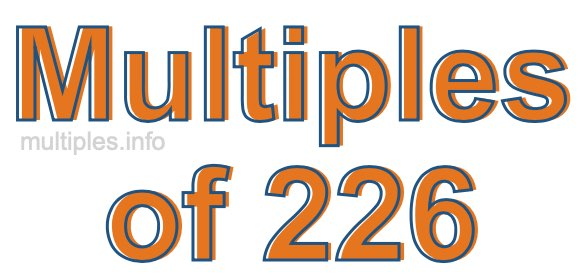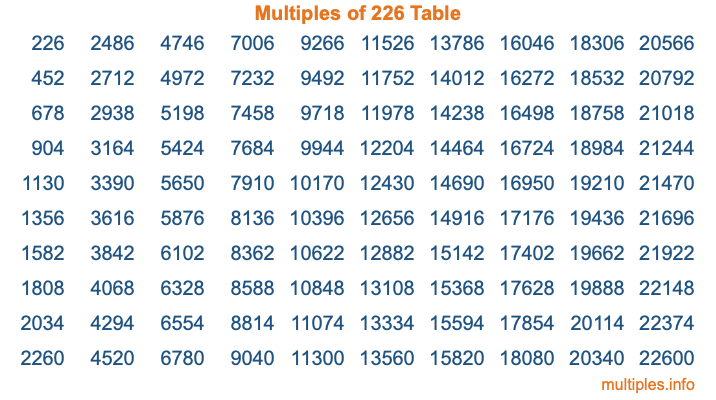Multiples of 226Welcome to the Multiples of 226 page. Here we will first teach you everything you will ever need to know about the multiples of 226, and then give you a study guide summary of everything we taught you to make sure you remember it all. Use this page to look up facts and learn information about the multiples of 226. This page will make you a multiples of two hundred twenty-six expert!

Definition of Multiples of 226
Multiples of 226 are all the numbers that when divided by 226 equal an integer. Each of the multiples of 226 are called a multiple. A multiple of 226 is created by multiplying 226 by an integer.

Therefore, to create a list of multiples of 226, you start with 1 multiplied by 226, then 2 multiplied by 226, then 3 multiplied by 226, and so on for as long as you want. Thus, the list of the first five multiples of 226 is 226, 452, 678, 904, and 1130. To see a larger list of multiples of 226, see the printable image of Multiples of 226 further down on this page. We also have a category where you can choose any nth multiple of 226.

Multiples of 226 Checker
The Multiples of 226 Checker below checks to see if any number of your choice is a multiple of 226. In other words, it checks to see if there is any number (integer) that when multiplied by 226 will equal your number. To do that, we divide your number by 226. If the the quotient is an integer, then your number is a multiple of 226.

Is  a multiple of 226?

Least Common Multiple of 226 and ...
A Least Common Multiple (LCM) is the lowest multiple that two or more numbers have in common. This is also called the smallest common multiple or lowest common multiple and is useful to know when you are adding our subtracting fractions. Enter one or more numbers below (226 is already entered) to find the LCM.

Check out our LCM Calculator if you need more details about the Least Common Multiple or if you need the LCM for different numbers for adding and subtraction fractions.

nth Multiple of 226
As we stated above, 226 is the first multiple of 226, 452 is the second multiple of 226, 678 is the third multiple of 226, and so on. Enter a number below to find the nth multiple of 226.

th multiple of 226

Multiples of 226 vs Factors of 226
226 is a multiple of 226 and a factor of 226, but that is where the similarities end. All postive multiples of 226 are 226 or greater than 226. All positive factors of 226 are 226 or less than 226.

Below is the beginning list of multiples of 226 and the factors of 226 so you can compare:

Multiples of 226: 226, 452, 678, 904, 1130, etc.

Factors of 226: 1, 2, 113, 226

As you can see, the multiples of 226 are all the numbers that you can divide by 226 to get a whole number. The factors of 226, on the other hand, are all the whole numbers that you can multiply by another whole number to get 226.

It's also interesting to note that if a number (x) is a factor of 226, then 226 will also be a multiple of that number (x).

Multiples of 226 vs Divisors of 226
The divisors of 226 are all the integers that 226 can be divided by evenly. Below is a list of the divisors of 226.

Divisors of 226: 1, 2, 113, 226

The interesting thing to note here is that if you take any multiple of 226 and divide it by a divisor of 226, you will see that the quotient is an integer.

Multiples of 226 Table
Below is an image of the first 100 multiples of 226 in a table. The table is in chronological order, column by column. The first column has the first ten multiples of 226, the second column has the next ten multiples of 226, and so on.The Multiples of 226 Table is also referred to as the 226 Times Table or Times Table of 226. You are welcome to print out our table for your studies.

Negative Multiples of 226
Although not often discussed or needed in math, it is worth mentioning that you can make a list of negative multiples of 226 by multiplying 226 by -1, then by -2, then by -3, and so on, to get the following list of negative multiples of 226:

-226, -452, -678, -904, -1130, etc.

Multiples of 226 Summary
Below is a summary of important Multiples of 226 facts that we have discussed on this page. To retain the knowledge on this page, we recommend that you read through the summary and explain to yourself or a study partner why they hold true.

There are an infinite number of multiples of 226.

A multiple of 226 divided by 226 will equal a whole number.

226 divided by a factor of 226 equals a divisor of 226.

The nth multiple of 226 is n times 226.

The largest factor of 226 is equal to the first positive multiple of 226.

226 is a multiple of every factor of 226.

226 is a multiple of 226.

A multiple of 226 divided by a divisor of 226 equals an integer.

226 divided by a divisor of 226 equals a factor of 226.

Any integer times 226 will equal a multiple of 226.

Multiples of a Number
Here you can get the multiples of another number, all with the same attention to detail as we did for multiples of 226 on this page.

Multiples of
Multiples of 227
Did you find our page about multiples of two hundred twenty-six educational? Do you want more knowledge? Check out the multiples of the next number on our list!